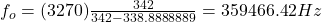## (a) At an air show a jet flies directly toward the stands at a speed of 1220 km/h, emitting a frequency of 3270 Hz, on a day when the speed

Question

(a) At an air show a jet flies directly toward the stands at a speed of 1220 km/h, emitting a frequency of 3270 Hz, on a day when the speed of sound is 342 m/s. What frequency (in Hz) is received by the observers?

in progress 0
2 months 2021-09-02T14:50:54+00:00 2 Answers 0 views 0

f’ = 358442.3 Hz

Explanation:

This is a problem about the Doppler’s effect. To find the perceived frequency of the observers you use the following formula: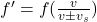v: speed of sound = 342 m/s

vs : speed of the source (jet) = 1220 km/h (1h/3600s)*(1000 m/ 1km) = 338.88 m/s  (it is convenient to convert the units of vs to m/s)

f: frequency emitted by the source = 3270 Hz

f’: perceived frequency

Due to the jet is getting closer to the observers, the sing of the denominator in equation (1) is minus (-). Then, you replace the values of f, vs and v: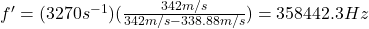Hence, the perceived frequency by the observers is 358442.3 Hz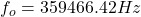Explanation:

You can solve this problem using doppler effect formula. The Doppler effect is the phenomenon by which the frequency of the waves perceived by an observer varies when the emitting focus or the observer itself moves relative to each other

Doppler effect general case is given by: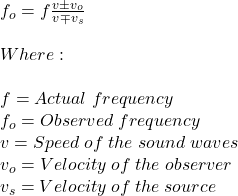Now, you can use the following facts: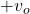Is used when the observer moves towards the source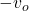Is used when the observer moves away from the source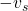Is used when the source moves towards the observer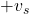Is used when the source moves away from the observer

Since the source is alone in motion towards the observer, the formula is given by: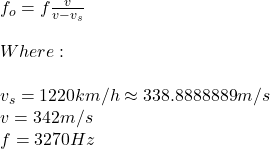Therefore: